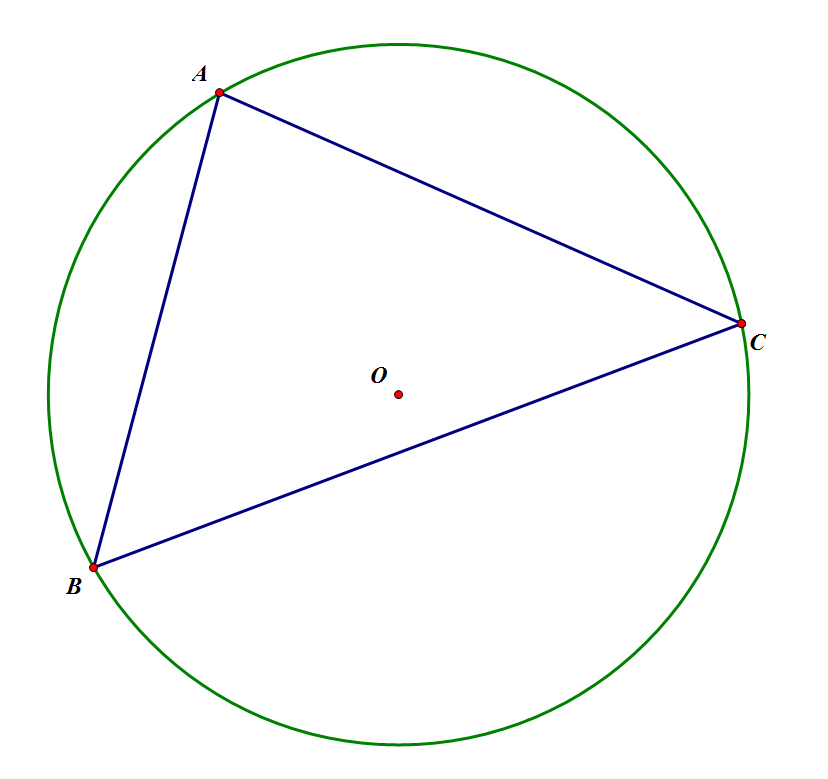Circle Areas #2: The Basics

Geometry Level 3In the diagram above, Circle O is circumscribed about Triangle ABC, where AB=$4$, AC=$4$, and BC=$6$. The area of Circle O can be expressed as $\frac { a\pi }{ b }$, where $a,b$ are constants, are positive integers, and share no common factors greater than 1. What is $a+b$?

Note: Not to scale

This is part of the set Circles, made by Chris H.

×